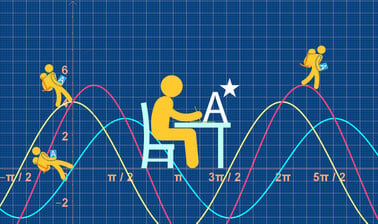# Imperial College London: A-level Mathematics for Year 13 - Course 1: Functions, Sequences and Series, and Numerical Methods

4.6 stars
5 ratings

Develop your thinking skills, fluency and confidence to aim for an A* in A-level maths and prepare for undergraduate STEM degrees.7 weeks
2–4 hours per week
Self-paced
Free

This course by Imperial College London is designed to help you develop the skills you need to succeed in your A-level maths exams.
The course is most appropriate to the Edexcel, AQA, OCR and OCR(MEI) papers. You will investigate key topic areas to gain a deeper understanding of the skills and techniques that you can apply throughout your A-level study.These skills include:

• Fluency – selecting and applying correct methods to answer with speed and efficiency
• Confidence – critically assessing mathematical methods and investigating ways to apply them
• Problem solving – analysing the ‘unfamiliar’ and identifying which skills and techniques you require to answer questions
• Constructing mathematical argument – using mathematical tools such as diagrams, graphs, logical deduction, mathematical symbols, mathematical language, construct mathematical argument and present precisely to others
• Deep reasoning – analysing and critiquing mathematical techniques, arguments, formulae and proofs to comprehend how they can be applied

Over seven modules, covering an introduction to functions and their notation, sequences and series and numerical methods testing your initial skillset will be extended to give a clear understanding of how background knowledge underpins the A-level course.

You’ll also be encouraged to consider how what you know fits into the wider mathematical world.

### At a glance

• Institution: ImperialX
• Subject: Math
• Level: Intermediate
• Prerequisites:
None
• Language: English
• Video Transcript: English
• Associated skills: Basic Math, Numerical Analysis

# What you'll learn

Skip What you'll learn

By the end of this course, you'll be able to:

• Define a mapping and a function
• Define the domain and range for a function
• Combine functions to create a composite function
• Find the inverse of a function
• Define a sequence using an nth term formula and an inductive definition
• Define an arithmetic and a geometric sequence
• Use sigma notation to define a series
• Expand a binomial expression for both a positive integer index and for an index which is not a positive integer
• Use radians as a measure of angle
• Find arc lengths, areas of sectors and areas of segments on circles where angles are in radians
• Use small angle approximations for sine, cosine and tangent functions
• Find multiple solutions to trigonometric equations
• Use the reciprocal trigonometric functions
• Use the inverse trigonometrical functions
• Use trigonometrical identities
• Derive and use the trapezium rule to find the area under a curve
• Approximate the root of an equation using a sign change method
• Approximate the root of an equation using the Newton-Raphson method
• Approximate the root of an equation by fixed point iteration

# Syllabus

Skip Syllabus

Module 1: Algebra and Functions

• The difference between a mapping and a function
• Function notation
• Domain and range of a function
• Composition of functions
• Inverse functions

Module 2: Sequences and Series 1

• How to define a sequence by an nth term rule
• How to define a sequence by an inductive rule
• Arithmetic sequences
• Geometric sequences
• The sum of n terms of an arithmetic and a geometric sequence
• Series and the sigma notation

Module 3: Sequences and Series 2

• The binomial expansion for positive integer n
• The general binomial expansion
• Properties of sequences

Module 4: Trigonometry 1

• Circle calculations
• Small angle approximations
• Circular functions

Module 5: Trigonometry 2

• The reciprocal trigonometric functions
• Inverse trigonometric functions
• Addition and double angle formulae
• Trigonometric identities

Module 6: Numerical Methods 1

• An introduction to numerical methods
• The trapezium rule
• Numerical solution of equations

Module 7: Numerical Methods 2

• The Newton-Raphson method
• Fixed point iteration